Physical Chemistry : Photons and Photon Energy

Example Questions

Example Question #1 : Photons And Photon Energy

Which of the following quantities is zero for photons?

Mass

Neither mass nor speed

Speed

Both mass and speed

Mass

Explanation:

Photons are fundamental particles that make up electromagnetic waves (light). The key aspect of photon is that it has very high speed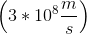and no mass; therefore, mass for photons is always zero.

Example Question #61 : Physical Chemistry

Which of the following is/are the same for photons in gamma rays and in visible light?

I. frequency

II. wavelength

III. speed

I only

I and II

III only

None of these

III only

Explanation:

Electromagnetic spectrum is a spectrum of different types of light. Each type of electromagnetic wave differs from one another based on the frequency and wavelength; however, the speed of every electromagnetic wave in air is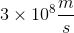. The wave equation relates the speed of an electromagnetic to its wavelength and frequency as follows.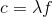Here,is speed of light,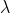is wavelength, andis frequency. Sinceis always constant, wavelength and frequency are inversely proportional to each other. This means that if the wavelength increases the frequency decreases.

Example Question #63 : Physical Chemistry

Photons in ultraviolet rays have a wavelength of 10nm. What is the energy of photons in radio waves?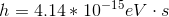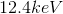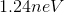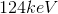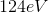Explanation:

To solve this question, we need to use the equation relating energy of a wave to its wavelength.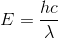Here,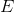is the energy,is Planck’s constant,is speed of light (for us it’s always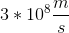), andis the wavelength (in meters). The energy of the ultraviolet rays is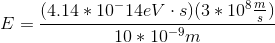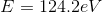If we look at the electromagnetic spectrum we will notice that the wavelength of radio waves is a lot higher than ultraviolet rays. Since energy is inversely proportional to wavelength we can conclude that the energy of radio waves is lower than ultraviolet's. The only answer choice with an energy lower than ultraviolet rays energy is 1.24neV.

All Physical Chemistry Resources